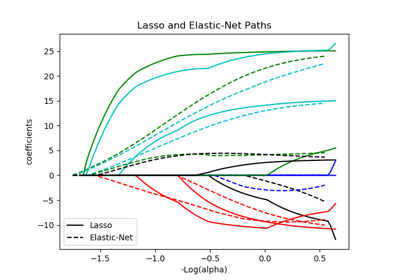# sklearn.linear_model.enet_path¶

sklearn.linear_model.enet_path(X, y, l1_ratio=0.5, eps=0.001, n_alphas=100, alphas=None, precompute='auto', Xy=None, copy_X=True, coef_init=None, verbose=False, return_n_iter=False, positive=False, check_input=True, **params)[source]

Compute elastic net path with coordinate descent.

The elastic net optimization function varies for mono and multi-outputs.

1 / (2 * n_samples) * ||y - Xw||^2_2
+ alpha * l1_ratio * ||w||_1
+ 0.5 * alpha * (1 - l1_ratio) * ||w||^2_2


(1 / (2 * n_samples)) * ||Y - XW||^Fro_2
+ alpha * l1_ratio * ||W||_21
+ 0.5 * alpha * (1 - l1_ratio) * ||W||_Fro^2


Where:

||W||_21 = \sum_i \sqrt{\sum_j w_{ij}^2}


i.e. the sum of norm of each row.

Read more in the User Guide.

Parameters
X{array-like}, shape (n_samples, n_features)

Training data. Pass directly as Fortran-contiguous data to avoid unnecessary memory duplication. If y is mono-output then X can be sparse.

yndarray, shape (n_samples,) or (n_samples, n_outputs)

Target values.

l1_ratiofloat, optional

Number between 0 and 1 passed to elastic net (scaling between l1 and l2 penalties). l1_ratio=1 corresponds to the Lasso.

epsfloat

Length of the path. eps=1e-3 means that alpha_min / alpha_max = 1e-3.

n_alphasint, optional

Number of alphas along the regularization path.

alphasndarray, optional

List of alphas where to compute the models. If None alphas are set automatically.

precomputeTrue | False | ‘auto’ | array-like

Whether to use a precomputed Gram matrix to speed up calculations. If set to 'auto' let us decide. The Gram matrix can also be passed as argument.

Xyarray-like, optional

Xy = np.dot(X.T, y) that can be precomputed. It is useful only when the Gram matrix is precomputed.

copy_Xbool, optional, default True

If True, X will be copied; else, it may be overwritten.

coef_initarray, shape (n_features, ) | None

The initial values of the coefficients.

verbosebool or int

Amount of verbosity.

return_n_iterbool

Whether to return the number of iterations or not.

positivebool, default False

If set to True, forces coefficients to be positive. (Only allowed when y.ndim == 1).

check_inputbool, default True

Skip input validation checks, including the Gram matrix when provided assuming there are handled by the caller when check_input=False.

**paramskwargs

Keyword arguments passed to the coordinate descent solver.

Returns
alphasarray, shape (n_alphas,)

The alphas along the path where models are computed.

coefsarray, shape (n_features, n_alphas) or (n_outputs, n_features, n_alphas)

Coefficients along the path.

dual_gapsarray, shape (n_alphas,)

The dual gaps at the end of the optimization for each alpha.

n_itersarray-like, shape (n_alphas,)

The number of iterations taken by the coordinate descent optimizer to reach the specified tolerance for each alpha. (Is returned when return_n_iter is set to True).

Notes

For an example, see examples/linear_model/plot_lasso_coordinate_descent_path.py.

## Examples using sklearn.linear_model.enet_path¶